Share

# Balbharati solutions for Maharashtra state board SSC 9th Standard Maths 2 Geometry chapter 6 - Circle [Latest edition]

Textbook page

#### Chapters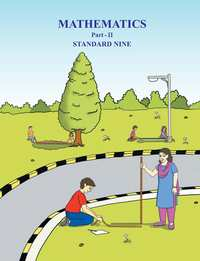## Chapter 6: Circle

Practice Set 6.1Practice Set 6.2Practice Set 6.3Problem Set 6Problem Set 6

### Balbharati solutions for Maharashtra state board SSC 9th Standard Maths 2 Geometry Chapter 6 Circle Practice Set 6.1 [Page 79]

Practice Set 6.1 | Q 1 | Page 79

Distance of chord AB from the centre of a circle is 8 cm. Length of the chord AB is 12 cm. Find the diameter of the circle.

Practice Set 6.1 | Q 2 | Page 79

Diameter of a circle is 26 cm and length of a chord of the circle is 24 cm. Find the distance of the chord from the centre.

Practice Set 6.1 | Q 3 | Page 79

Radius of a circle is 34 cm and the distance of the chord from the centre is 30 cm, find the length of the chord.

Practice Set 6.1 | Q 4 | Page 79

Radius of a circle with centre O is 41 units. Length of a chord PQ is 80 units, find the distance of the chord from the centre of the circle.

Practice Set 6.1 | Q 5 | Page 79

In the given figure, centre of two circles is O. Chord AB of bigger circle intersects the smaller circle in points P and Q. Show that AP = BQ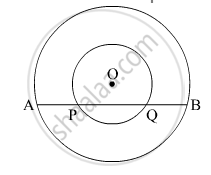Practice Set 6.1 | Q 6 | Page 79

Prove that, if a diameter of a circle bisects two chords of the circle then those two chords are parallel to each other.

### Balbharati solutions for Maharashtra state board SSC 9th Standard Maths 2 Geometry Chapter 6 Circle Practice Set 6.2 [Page 82]

Practice Set 6.2 | Q 1 | Page 82

Radius of circle is 10 cm. There are two chords of length 16 cm each. What will be the distance of these chords from the centre of the circle ?

Practice Set 6.2 | Q 2 | Page 82

In a circle with radius 13 cm, two equal chords are at a distance of 5 cm from the centre. Find the lengths of the chords.

Practice Set 6.2 | Q 3 | Page 82

Seg PM and seg PN are congruent chords of a circle with centre C. Show that the ray PC is the bisector of ∠ NPM.

### Balbharati solutions for Maharashtra state board SSC 9th Standard Maths 2 Geometry Chapter 6 Circle Practice Set 6.3 [Page 86]

Practice Set 6.3 | Q 1 | Page 86

Construct Δ ABC such that ∠B = 100° , BC = 6.4 cm, ∠C = 50° and construct its incircle.

Practice Set 6.3 | Q 2 | Page 86

Construct Δ PQR such that ∠P = 70° , ∠R = 50° , QR = 7.3 cm  and constructs its circumcircle.

Practice Set 6.3 | Q 3 | Page 86

Construct Δ XYZ such that XY = 6.7 cm ,YZ = 5.8 cm, XZ = 6.9 cm  and constructs its incircle.

Practice Set 6.3 | Q 4 | Page 86

In Δ LMN,  LM = 7.2 cm, ∠M = 105° , MN = 6.4 cm , then draw Δ LMN   and construct  its circumcircle.

Practice Set 6.3 | Q 5 | Page 86

Construct Δ  DEF  such that   DE = EF = 6 cm, ∠ F = 45°  and constructs its circumcircle.

### Balbharati solutions for Maharashtra state board SSC 9th Standard Maths 2 Geometry Chapter 6 Circle Problem Set 6, Problem Set 6 [Pages 86 - 87]

Problem Set 6 | Q 1.1 | Page 86

Choose correct alternative answer and fill in the blank.

Radius of a circle is 10 cm and distance of a chord from the centre is 6 cm. Hence the length of the chord is .........

• 16 cm

• 8 cm

• 12 cm

• 32 cm

Problem Set 6 | Q 1.2 | Page 86

Choose correct alternative answer and fill in the blank.

The point of concurrence of all angle bisectors of a triangle is called the ......

• centroid

• circumcentre

• incentre

• orthocentre

Problem Set 6 | Q 1.3 | Page 86

Choose correct alternative answer and fill in the blank.

The circle which passes through all the vertices of a triangle is called .....

• circumcircle

• incircle

• congruent circle

• concentric circle

Problem Set 6 | Q 1.4 | Page 86

Choose correct alternative answer and fill in the blank.

Length of a chord of a circle is 24 cm. If distance of the chord from the centre is 5 cm, then the radius of that circle is ....

• 12 cm

• 13 cm

• 14 cm

• 15 cm

Problem Set 6 | Q 1.5 | Page 86

Choose correct alternative answer and fill in the blank.

The length of the longest chord of the circle with radius 2.9 cm is .....

• 3.5 cm

• 7 cm

• 10 cm

• 5.8 cm

Problem Set 6 | Q 1.6 | Page 86

Choose correct alternative answer and fill in the blank.

Radius of a circle with centre O is 4 cm. If l(OP) = 4.2 cm, say where point P will lie.

• on the centre

• Inside the circle

• outside the circle

• on the circle

Problem Set 6 | Q 1.7 | Page 86

Choose correct alternative answer and fill in the blank.

The lengths of parallel chords which are on opposite sides of the centre of a circle are 6 cm and 8 cm. If radius of the circle is 5 cm, then the distance between these chords is .....

• 2 cm

• 1 cm

• 8 cm

• 7 cm

Problem Set 6 | Q 2 | Page 87

Construct incircle and circumcircle of an equilateral
Δ DSP with side 7.5 cm. Measure the radii of both the circles and find the ratio of radius of circumcircle to the radius of incircle.

Problem Set 6 | Q 3 | Page 87

Construct Δ NTS where NT = 5.7 cm, TS = 7.5 cm and
∠ NTS = 110 ° and draw incircle  and circumcircle of it.

Problem Set 6 | Q 4 | Page 87

In the given figure, C is the centre of the circle. seg QT is a diameter CT = 13, CP = 5, find the length of chord RS.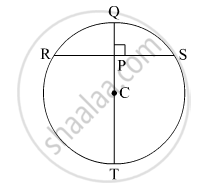Problem Set 6 | Q 5 | Page 87
In the given figure, P is the centre of the circle. chord AB and chord CD intersect on the diameter at the point E
If ∠ AEP ≅ ∠ DEP  then prove that AB = CD.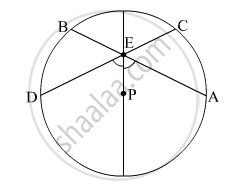Problem Set 6 | Q 6 | Page 87

In the given figure, CD is a diameter of the circle with centre O. Diameter CD is perpendicular to chord AB at point E. Show that Δ ABC is an isosceles triangle.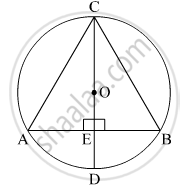## Chapter 6: Circle

Practice Set 6.1Practice Set 6.2Practice Set 6.3Problem Set 6Problem Set 6## Balbharati solutions for Maharashtra state board SSC 9th Standard Maths 2 Geometry chapter 6 - Circle

Balbharati solutions for Maharashtra state board SSC 9th Standard Maths 2 Geometry chapter 6 (Circle) include all questions with solution and detail explanation. This will clear students doubts about any question and improve application skills while preparing for board exams. The detailed, step-by-step solutions will help you understand the concepts better and clear your confusions, if any. Shaalaa.com has the Maharashtra State Board Maharashtra state board SSC 9th Standard Maths 2 Geometry solutions in a manner that help students grasp basic concepts better and faster.

Further, we at Shaalaa.com provide such solutions so that students can prepare for written exams. Balbharati textbook solutions can be a core help for self-study and acts as a perfect self-help guidance for students.

Concepts covered in Maharashtra state board SSC 9th Standard Maths 2 Geometry chapter 6 Circle are Concept of Circles, Properties of Chord, Incircle Of a triangle, Circumcircle of circle.

Using Balbharati Class 9 solutions Circle exercise by students are an easy way to prepare for the exams, as they involve solutions arranged chapter-wise also page wise. The questions involved in Balbharati Solutions are important questions that can be asked in the final exam. Maximum students of Maharashtra State Board Class 9 prefer Balbharati Textbook Solutions to score more in exam.

Get the free view of chapter 6 Circle Class 9 extra questions for Maharashtra state board SSC 9th Standard Maths 2 Geometry and can use Shaalaa.com to keep it handy for your exam preparation